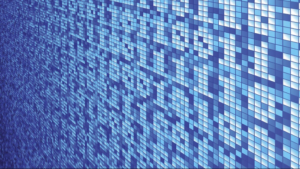## Putting It Together: ExponentsBits and Bytes

Exponents allow us to perform repeated multiplication quickly and to write very large or small numbers in a way that is easy to understand. There are many and varied uses of exponents that you may be surprised to find are all around us.  Have you ever heard a computer described as having a $32$-bit processor with $64$ megabytes of RAM and $2.1$ gigabytes of hard disk space?  What on earth does that even mean? This terminology is used to describe how fast a computer can process information, which results in how quickly it responds after you click on an app or open a file. It also describes how much memory the computer has to store the files and apps you want to use with it.

Computers use binary digits ($0$ and$1$) instead of the decimal digits ($0$ to $9$) we are used to working with. The descriptions $32$-bit and $64$ megabytes don’t make much sense at first glance, but you can use the lingua franca of exponents to translate this information into an explanation of how memory and processing speed work.

Each binary digit is equivalent to one bit, and a byte contains $8$ bits, often called a binary octet. Each character that you type on a computer needs one binary octet in order to exist. This is what a byte represents relative to decimal numbers:

 Type of Number Decimal Equivalent Decimal Powers of 2 $2^7$ $2^6$ $2^5$ $2^4$ $2^3$ $2^2$ $2^1$ $2^0$ Decimal Number $128$ $64$ $32$ $16$ $8$ $4$ $2$ $1$ $128+64+32+16+8+4+2+1=255$ Binary Octet $0$ $0$ $0$ $0$ $0$ $0$ $0$ $1$ $1$ $0$ $0$ $0$ $0$ $0$ $0$ $1$ $1$ $2+1=3$ $0$ $0$ $0$ $0$ $1$ $1$ $1$ $0$ $8+4+2=14$ $1$ $0$ $1$ $0$ $1$ $1$ $0$ $0$ $128+32+8+4=172$

The $1$s in the binary octet act as light switches within a computer. The computer uses the binary octets to represent characters such as numbers, letters, and other information.  You can think of the number of bits assigned to a computer processor as the amount of “scratch pad” it has available to store calculations and information while it works.  Having more “scratch pad” available means the computer can do more without needing to access memory which takes time.  There are many more factors that influence the speed and agility of a computer, but the basics can be described with exponents. Now try to imagine how many bytes the Millennium Falcon’s computer must have needed to make the Kessel Run in less than $12$ parsecs!

## Contribute!

Did you have an idea for improving this content? We’d love your input.